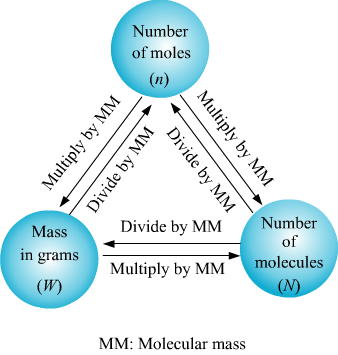Mole Concept Calculation

This article will discuss about few basics and fundamental calculation of mole concept, which is of utmost importance in solving any kind of problem-related to mole concept.

Conversion of moles to mass and vice-versaThe key concept used in these kind of problems is that a mole of any substance contains gram formula mass or molecular mass of that substance i.e. molecular mass of Hydrogen is 2 a.m.u. so mass of 1 mole of hydrogen which is also known as molar mass will be 2 gram. Similarly if we have 2 moles of hydrogen, it will weigh 2*2 grams which is equal to 4 grams.

Example

Question – Two containers A & B are filled with 4 gram hydrogen gas and 32 gram oxygen gas respectively. Which of the two containers have more moles?

Solution:-

Molecular mass of Hydrogen is 2, and that of Oxygen is 32

2 gram of hydrogen means 1 mole of hydrogen gas (H2)

Or, 4 gram of hydrogen means (½)*4 mole of hydrogen

= 2 moles of hydrogen

Similarly, 32 grams of oxygen gas (O2) means 1 mole of gas.

As clear from the above calculation, container A (filled with 4 gram hydrogen gas) will have more number of moles than container B (filled with 32 grams oxygen gas).

Mole concept calculation

This is the most basic and the most used calculation that a student comes across while solving a mole concept problem. Most of the times, moles or number of atoms or molecules are given in the question and the mass is needed to be calculated. In that case proceed as shown in the above example. In rest of the cases, mass will be given and moles or number will be needed to be calculated. In those questions also, proceed by:

STEP 1:- Establishing relationship between molar mass and the number (NA) or moles of that particular entity (atom, molecule or ion).

STEP 2:- Use unitary method to calculate what is asked in the question.

NOTE: – When we say oxygen gas weighs 32 gram then we mean to say that 1 mole of oxygen molecule (O2) weighs 32 grams and not 1 mole of oxygen atom which is O. This is because in natural form, oxygen exists as O2 molecule.